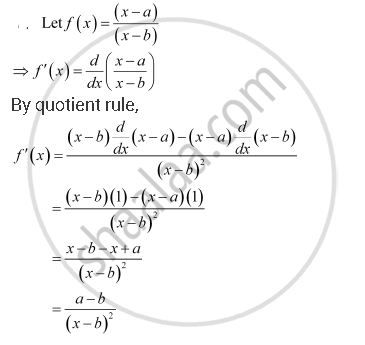CBSE (Arts) Class 11CBSE
Share

Books Shortlist

For Some Constants A And B, Find the Derivative of (X-a)/(X -b) - CBSE (Arts) Class 11 - Mathematics

ConceptDerivative Derivative of Polynomials and Trigonometric Functions

Question

For some constants a and b, find the derivative of

(x-a)/(x -b)

SolutionIs there an error in this question or solution?

APPEARS IN

NCERT Solution for Mathematics Textbook for Class 11 (2013 to Current)
Chapter 13: Limits and Derivatives
Q: 7.3 | Page no. 313
Solution For Some Constants A And B, Find the Derivative of (X-a)/(X -b) Concept: Derivative - Derivative of Polynomials and Trigonometric Functions.
S